Examples

Chapter 9 Class 11 Sequences and Series (Term 1)
Serial order wise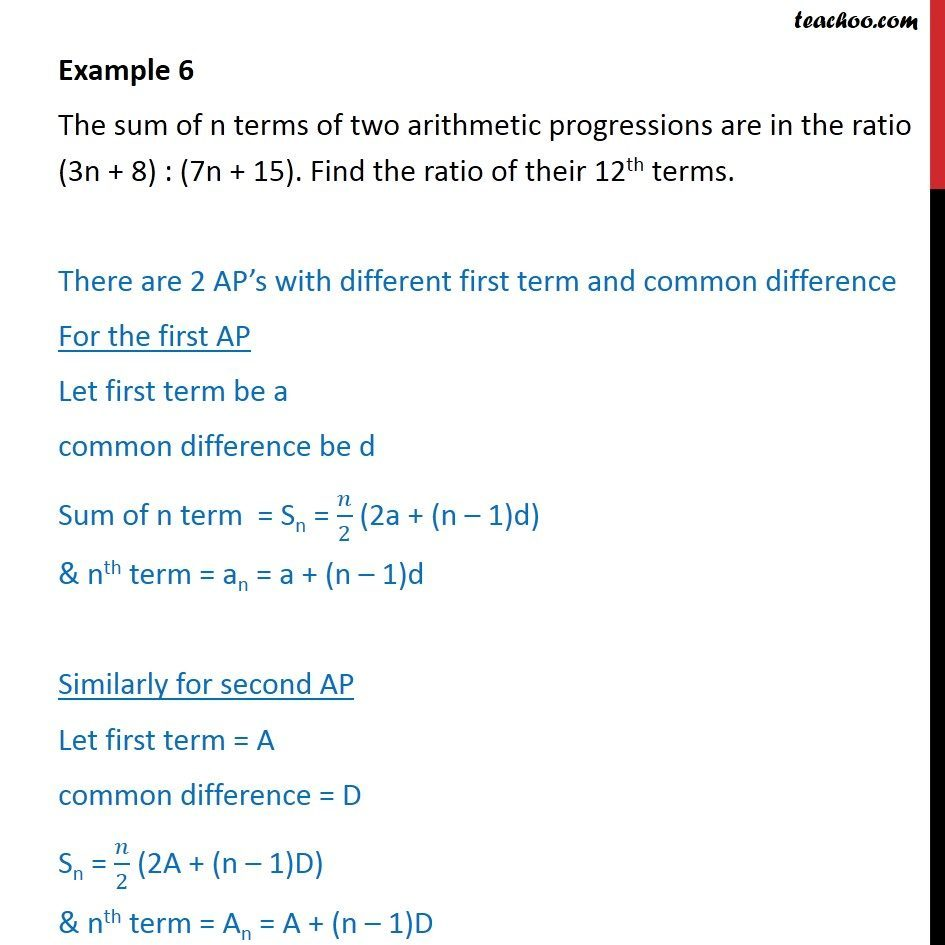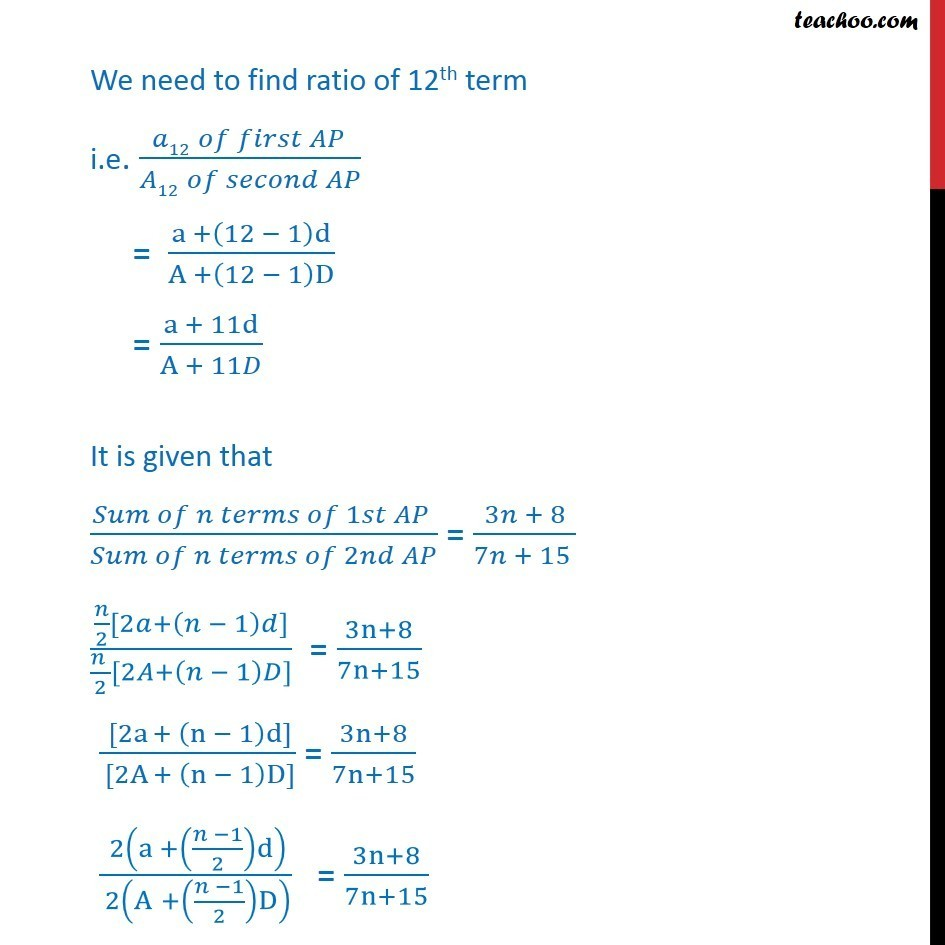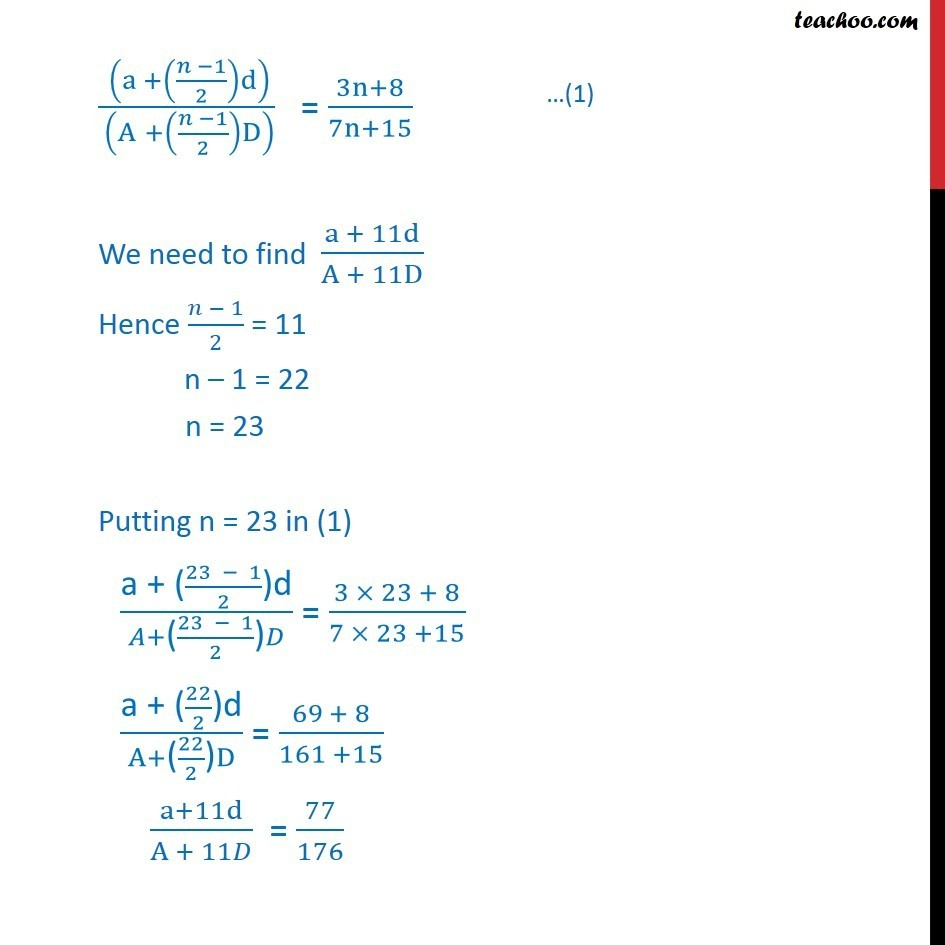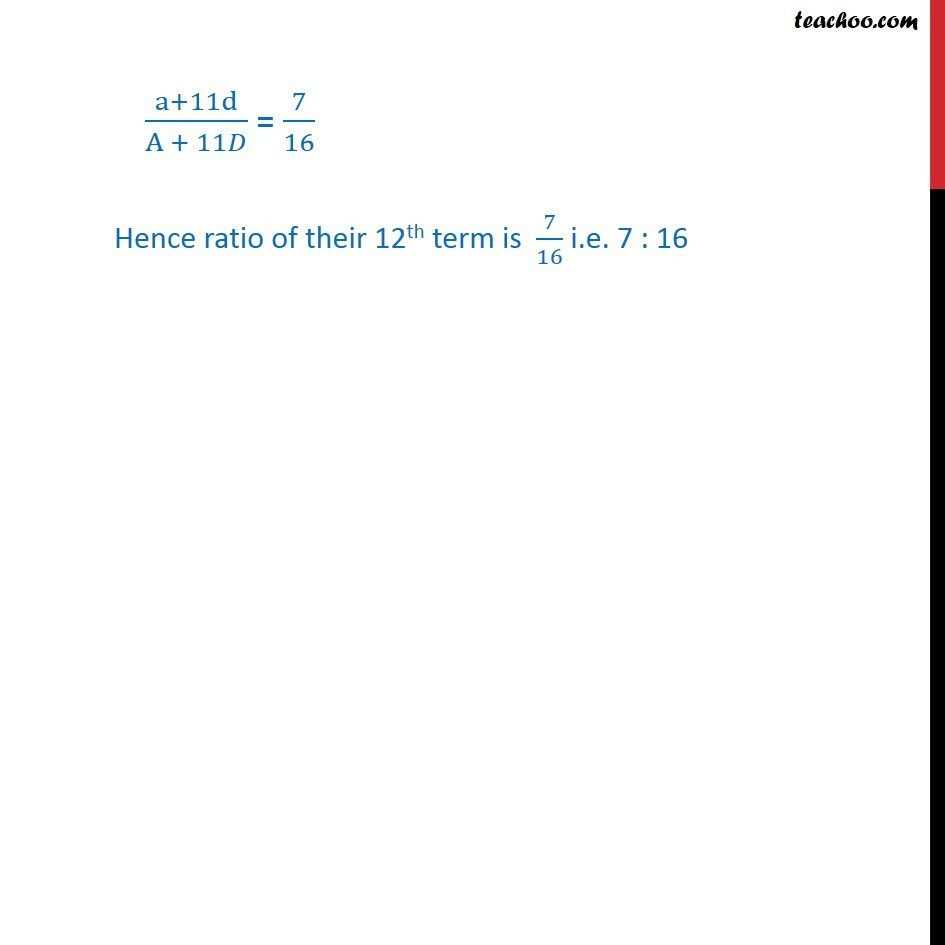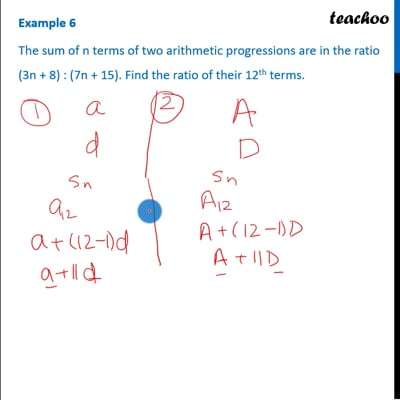This video is only available for Teachoo black users

### Transcript

Example 6 The sum of n terms of two arithmetic progressions are in the ratio (3n + 8) : (7n + 15). Find the ratio of their 12th terms. There are 2 AP s with different first term and common difference For the first AP Let first term be a common difference be d Sum of n term = Sn = /2 (2a + (n 1)d) & nth term = an = a + (n 1)d Similarly for second AP Let first term = A common difference = D Sn = /2 (2A + (n 1)D) & nth term = An = A + (n 1)D We need to find ratio of 12th term i.e. ( 12 )/( 12 ) = (a +(12 1)d)/(A +(12 1)D) = (a + 11d)/(A + 11 ) It is given that ( 1 )/( 2 ) = (3 + 8)/(7 + 15 ) ( /2[2 +( 1) ])/(( )/2[2 +( 1) ]) = (3n+8)/(7n+15) ( [2a + (n 1)d])/( [2A + (n 1)D]) = (3n+8)/(7n+15) ( 2(a +(( 1)/2)d))/( 2(A +(( 1)/2)D) ) = (3n+8)/(7n+15) ( (a +(( 1)/2)d))/( (A +(( 1)/2)D) ) = (3n+8)/(7n+15) We need to find (a + 11d)/(A + 11D) Hence ( 1)/2 = 11 n 1 = 22 n = 23 Putting n = 23 in (1) ("a + (" (23 1)/2 ")d" )/( +"(" (23 1)/2 ")" ) = (3 23 + 8)/(7 23 +15) ("a + (" 22/2 ")d" )/(A+"(" 22/2 ")" D) = (69 + 8)/(161 +15) (a+11d)/(A + 11 ) = 77/176 (a+11d)/(A + 11 ) = 7/16 Hence ratio of their 12th term is 7/16 i.e. 7 : 16